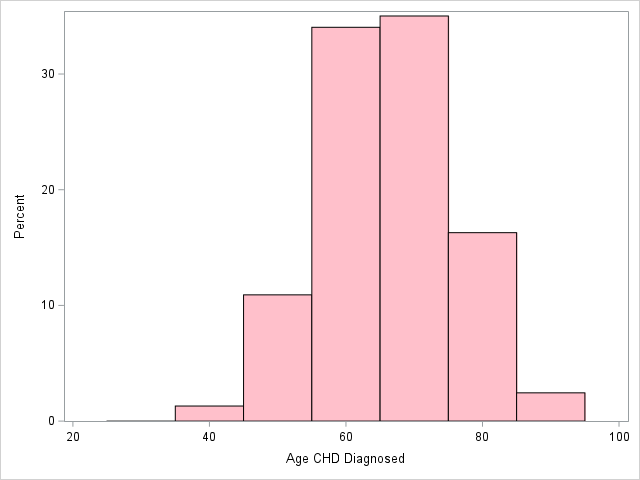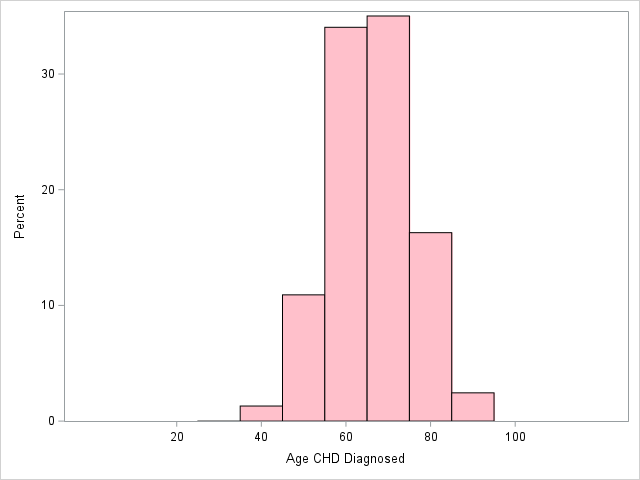## Change barwidth sgplot histogram

Hi all,

is there any way to change the barwidth

in sgplot histogram statement?

1 ACCEPTED SOLUTION

Accepted Solutions

## Re: Change barwidth sgplot histogram

If I understand your question, you want to control the physical width of the bars (not the 'width' of the data bin - ie, not the range of values in each bar) ? ...

In sgplot histograms, I believe the physical width of the bars expands to fill most/all available space. Therefore one way to control the physical width of the bars would be to change the width of the page. (But you probably don't want that.)

You can also add more 'offset' between the bars and the axes, using the xaxis offsetmin= and offsetmax= options, to 'squeeze' the bars into a smaller space and make them 'skinnier' (offsetmin controls the space on the left side, and offsetmax controls the right side). Here's an example, without and with the offsetmin and offsetmax options:

/* default, with no offsetmin/offsetmax specified */

proc sgplot data=sashelp.heart;
where sex=:'F';
histogram agechddiag / nbins=7 fillattrs=(color=pink);
run;proc sgplot data=sashelp.heart;
where sex=:'F';
histogram agechddiag / nbins=7 fillattrs=(color=pink);
xaxis offsetmin=.2 offsetmax=.2;
run;12 REPLIES 12

## Re: Change barwidth sgplot histogram

Hi thanks, is binwidth=barwidth. This is not giving me the right results, even if I use even when I use

binwidth= binstart=  showbins

## Re: Change barwidth sgplot histogram

@Anita_n wrote:

Hi thanks, is binwidth=barwidth. This is not giving me the right results, even if I use even when I use

binwidth= binstart=  showbins

Explain further. What is not giving you the right results? What isn't right about them? Show us your code. Show us your output.

--
Paige Miller

## Re: Change barwidth sgplot histogram

``````
proc sgplot data=sashelp.heart;
where sex=:'F';
histogram agechddiag / fillattrs=(color=pink);
run;

proc sgplot data=sashelp.heart;
where sex=:'F';
histogram agechddiag / fillattrs=(color=pink) binwidth=5 binstart=50 showbins;
run;``````

## Re: Change barwidth sgplot histogram

Please explain what is wrong with the plot, and what you would like to see.

--
Paige MillerKsharp
Super User

## Re: Change barwidth sgplot histogram

```ods select histogram;
proc univariate data=sashelp.heart ;
where sex=:'F';
var agechddiag ;
histogram agechddiag / kernel  barwidth=1;
run;

proc univariate data=sashelp.heart ;
where sex=:'F';
var agechddiag ;
histogram agechddiag / kernel  endpoints=(30 to 100 by 20)  ;
run;

```Ksharp
Super User

## Re: Change barwidth sgplot histogram

``````

proc sgplot data=sashelp.heart;
where sex=:'F';
histogram agechddiag / fillattrs=(color=pink) nbins=10  showbins;
run;

proc sgplot data=sashelp.heart;
where sex=:'F';
histogram agechddiag / fillattrs=(color=pink) nbins=5  showbins;
run;``````

## Re: Change barwidth sgplot histogram

what does this nbins really do? I just wanted to reduce the size of the bars like when using barwidth=0.9 or barwidth=0.6DanH_sas
SAS Super FREQ

## Re: Change barwidth sgplot histogram

In a histogram, the bar width is data-driven. The width of the bar shows the range of values included in the bin. By changing the number of bins (NBINS), you change the range of values in each bin. The more bins you have, the thinner the bars will be.

## Re: Change barwidth sgplot histogram

Okay , thanks I now understand how it works

## Re: Change barwidth sgplot histogram

If I understand your question, you want to control the physical width of the bars (not the 'width' of the data bin - ie, not the range of values in each bar) ? ...

In sgplot histograms, I believe the physical width of the bars expands to fill most/all available space. Therefore one way to control the physical width of the bars would be to change the width of the page. (But you probably don't want that.)

You can also add more 'offset' between the bars and the axes, using the xaxis offsetmin= and offsetmax= options, to 'squeeze' the bars into a smaller space and make them 'skinnier' (offsetmin controls the space on the left side, and offsetmax controls the right side). Here's an example, without and with the offsetmin and offsetmax options:

/* default, with no offsetmin/offsetmax specified */

proc sgplot data=sashelp.heart;
where sex=:'F';
histogram agechddiag / nbins=7 fillattrs=(color=pink);
run;proc sgplot data=sashelp.heart;
where sex=:'F';
histogram agechddiag / nbins=7 fillattrs=(color=pink);
xaxis offsetmin=.2 offsetmax=.2;
run;## Re: Change barwidth sgplot histogram

@GraphGuy yes, that is exactly what I wanted. Thanks

Discussion stats
• 12 replies
• 2984 views
• 5 likes
• 5 in conversation